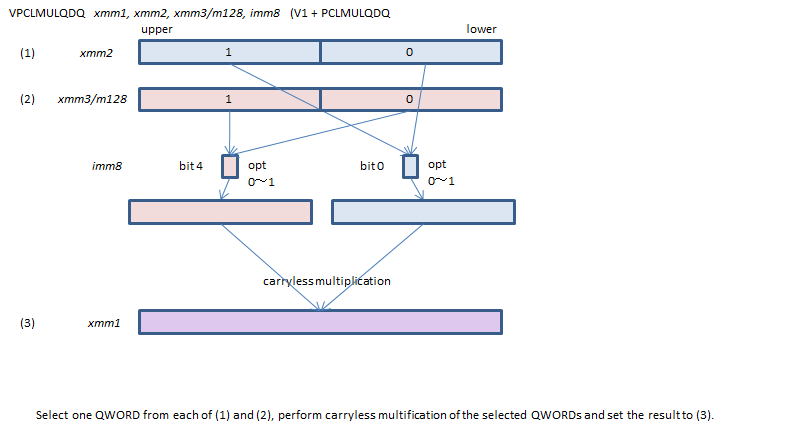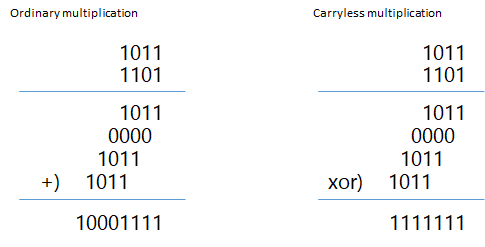﻿ pclmulqdq

## PCLMULQDQ - Packed CarryLess MULtiplication Qword DoubleQwordCarryless multiplication is a special operation used in some cipher algorithms.## Sample: applying to GCM/GMAC dot operation

In the Intel sample, this instruction is used only for the calculation of the first 255-bit product, and subsequent multiplications with constant R are done by combinations of bit shifts and XORs. But using this instruction more extensively can accelerate the execution (at least on my environment: Visiaul Studio 2017 + i7-7700).

```/////////////////////////////////////////////////
// Dot operation for GCM/GMAC
// NOTE: x, y and return value are LITTLE ENDIAN

// defining this constant within the function slows down the execution incredibly
// in MS Visual Studio Envrironment.
static const __m128i R = _mm_set_epi64x(0xE100000000000000, 0);

__m128i GCM_Mul_LE(__m128i x, __m128i y)
{
// CLMUL x and y to produce the 255-bit product z:zL

__m128i zL  = _mm_clmulepi64_si128(x, y, 0x00);
__m128i zM  = _mm_clmulepi64_si128(x, y, 0x01);
__m128i zM2 = _mm_clmulepi64_si128(x, y, 0x10);
__m128i z   = _mm_clmulepi64_si128(x, y, 0x11);
zM = _mm_xor_si128(zM, zM2);
zL = _mm_xor_si128(zL, _mm_slli_si128(zM, 8));
z  = _mm_xor_si128(z,  _mm_srli_si128(zM, 8));

// z:zL 256-bit data
//  bit 255: zero
//  bit 254-127: the upper 128-bit of the product
//  bit 126-0: overflow bits

// Extract the overflow bits, aligned to the left.
__m128i ov = _mm_slli_epi64(zL, 1);
ov = _mm_or_si128(ov, _mm_slli_si128(_mm_srli_epi64(zL, 63), 8));

// CLMUL lower part of overflow bits and R.
__m128i wM = _mm_clmulepi64_si128(ov, R, 0x10);
__m128i wL = _mm_slli_si128(wM, 8);

// wL 128-bit data
//  bit 127: the LSB of the upper 128-bit of R * the overflow bits
//  bit 126-121: the overflow-again bits
//  bit 120-0: zero

// Extract the overflow-again bits,  aligned to the left.
__m128i w2 = _mm_slli_epi64(wL, 1);
ov = _mm_xor_si128(ov, w2);

// CLMUL (the overflow bits ^ the overflow-again bits) and R.
__m128i w  = _mm_clmulepi64_si128(ov, R, 0x11);
// wM and wL is not affected by the XORing of the overflow-again bits
// so we can use the values calculated before.
w = _mm_xor_si128(w, _mm_srli_si128(wM, 8));

// w:wL 256-bit data
//  bit 255: zero
//  bit 254-127: the result of R * (the overflow bits ^ the overflow-again bits)
//  bit 126-0: garbage

// xor to make the final result.
z  = _mm_xor_si128(z, w);
zL = _mm_xor_si128(zL, wL);

// z:zL 256-bit data
//  bit 255: zero
//  bit 254-127: The final result
//  bit 126-0: garbage

// Extract the final result.
__m128i hc = _mm_srli_epi64(z, 63);
__m128i lc = _mm_srli_epi64(zL, 63);
z  = _mm_slli_epi64(z, 1);
hc = _mm_unpackhi_epi64(lc, _mm_slli_si128(hc, 8));
z  = _mm_or_si128(z, hc);

return z;
}
```

x86/x64 SIMD Instruction List  Feedback